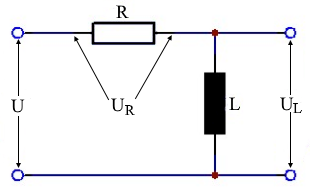# Resistor and inductor in series

Calculator and formulas for calculating resistor and inductor in series

## Calculator for RL series circuit

This function calculates the voltages, power, current, impedance and reactance for a resistor and inductor in series.

RL series circuit calculator

 Input Coil H mH µH nH Resistor mΩ Ω kΩ MΩ Frequency Hz kHz MHz GHz Voltage mV V kV Decimal places 0 1 2 3 4 6 8 10 Results Resistance XL Impedance Z Voltage UR Voltage UL Current I Real power P Reactive power Q Apparent power S Phase angle φ$$\displaystyle U$$ Applied voltage $$\displaystyle U_L$$ Voltage on the coil $$\displaystyle U_R$$ Voltage across the resistor $$\displaystyle I$$ Current $$\displaystyle R$$ Ohmic resistance $$\displaystyle X_L$$ Inductive reactance $$\displaystyle Z$$ Impedance $$\displaystyle S$$ Apparent power $$\displaystyle Q$$ Inductive reactive power $$\displaystyle p$$ Real power $$\displaystyle φ$$ Phase shift in °

## Formulas and description for RL in series

The total resistance of the RL series in the AC circuit is referred to as the impedance Z. Ohm's law applies to the entire circuit.

The current is the same at every measuring point. Current and voltage are in phase at the ohmic resistance. In the inductive reactance of the coil the current lag the voltage by −90 °.

The total voltage U is the sum of the geometrically added partial voltages. For this purpose, both partial voltages form the legs of a right triangle. Its hypotenuse corresponds to the total voltage U. The resulting triangle is called the voltage triangle or vector diagram of the voltages.

### Voltage triangle

 $$\displaystyle U=\sqrt{ {U_R}^2 + {U_L}^2}$$ $$\displaystyle U_R=\sqrt{U^2-{U_L}^2}$$ $$\displaystyle =U·cos(φ)$$ $$\displaystyle U_L=\sqrt{U^2 - {U_R}^2}$$ $$\displaystyle =U · sin(φ)$$ $$\displaystyle φ =arctan\left( \frac{X_L}{R} \right)$$

### Resistance triangle

 $$\displaystyle Z=\sqrt{R^2 + {X_L}^2}$$ $$\displaystyle =\frac{U}{I}$$ $$\displaystyle R=\sqrt{Z^2-{X_L}^2}$$ $$\displaystyle =\frac{U_R}{I}$$ $$\displaystyle =Z·cos(φ)$$ $$\displaystyle X_L=\sqrt{Z^2-R^2}$$ $$\displaystyle =\frac{U_L}{I}$$ $$\displaystyle =Z·sin(φ)$$ $$\displaystyle φ =arctan\left( \frac{U_L}{U_R} \right)$$

### Power triangle

 $$\displaystyle S=\sqrt{P^2 + Q^2}$$ $$\displaystyle =U·I$$ $$\displaystyle P=\sqrt{S^2-Q^2}$$ $$\displaystyle =U_R· I$$ $$\displaystyle =S·cos(φ)$$ $$\displaystyle Q=\sqrt{S^2-P^2}$$ $$\displaystyle = U_L· I$$ $$\displaystyle =S·sin(φ)$$

### Power factor

$$\displaystyle cos(φ)=\frac{P}{s}=\frac{U_R}{U}=\frac{R}{Z}$$

 Ist diese Seite hilfreich?             Vielen Dank für Ihr Feedback! Wie können wir die Seite verbessern?